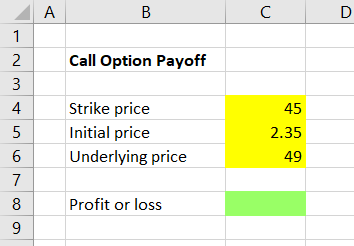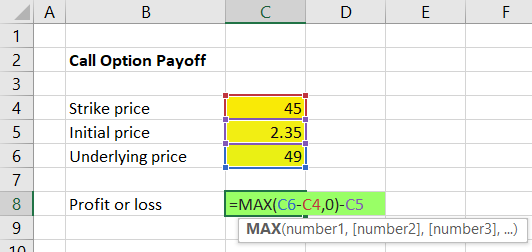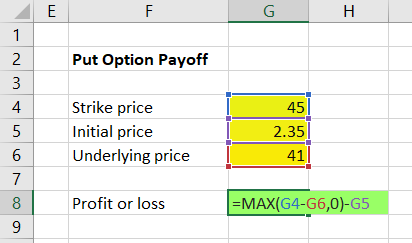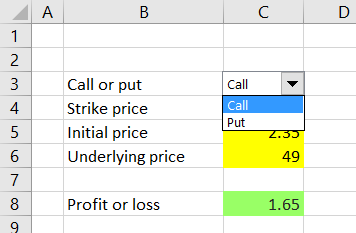# Calculating Call and Put Option Payoff in Excel

This is the first part of the Option Payoff Excel Tutorial. In this part we will learn how to calculate single option (call or put) profit or loss for a given underlying price. This is the basic building block that will allow us to calculate profit or loss for positions composed of multiple options, draw payoff diagrams in Excel, and calculate risk-reward ratios and break-even points.

## Understanding Option Payoff Formulas

Before we start building the actual formulas in Excel, let's make sure we understand what an option payoff formula is: It is a function that calculates how much money we make or lose at a particular underlying price.

For example, it answers the following question:

I have bought a \$45 strike call option for \$2.35. What will my profit or loss be if the underlying ends up at \$49 at expiration?

## Payoff Formula Inputs and Outputs

In the above example you can identify several inputs that our payoff formula will take – they are the numbers we already know:

1. Strike price of the option = 45
2. Initial price for which we have bought the option = 2.35
3. Underlying price for which we want to calculate the profit or loss = 49

The output is the profit or loss that we want to calculate.

## Preparing the Cells

In an Excel spreadsheet, we first need to set up three cells where we will enter the inputs, and another cell which will show the output.I have decided to enter the strike, initial price and underlying price inputs in cells C4, C5, C6, respectively. The result will be shown in cell C8.

While not necessary for a simple calculation like this one, it is a good idea to somehow graphically differentiate input and output cells, especially when building a more complex spreadsheet. It will make the sheet much easier to use and reduce the risk of you or someone else accidentally overwriting formulas in the future. It is best to do this consistently across all your spreadsheets. Personally, I always make the background of input cells (where user is expected to enter values) yellow and the output cells (which typically contain formulas and should not be overwritten) green – just my habit; you can of course use different colors, fonts, borders, or other formatting.

## Call Option Value Formula

Now we have the cells ready and we can build the formula in cell C8, which will use the inputs in the other cells to calculate profit or loss.

In general, call option value (not profit or loss) at expiration at a given underlying price is equal to the greater of:

• underlying price minus strike price (if the option expires in the money)
• zero (if it doesn't)

If you don't understand why, see detailed explanation and examples in Call Option Payoff Diagram, Formula and Logic.

Now we need to implement this formula in Excel. It is very easy, because Excel has the MAX function, which takes a set of values (separated with commas) and returns the greatest of them. In our example, the formula in cell C8 will be:

``=MAX(C6-C4,0)``

... where cells C4 and C6 are strike price and underlying price, respectively.With the inputs in our example (45 and 49), cell C8 should now be showing 4. You can test different values for the underlying price input and see how the formula works. For any underlying price smaller than or equal to 45 it should return zero; for values greater than 45 it should return the difference between cells C6 and C4.

But we are not finished yet.

## Call Option Profit or Loss Formula

Because we want to calculate profit or loss (not just the option's value), we must subtract our initial cost. This is again very simple to do – we will just subtract cell C5 from the result in cell C8. The entire formula in C8 becomes:

``=MAX(C6-C4,0)-C5``Cell C8 should now be showing 1.65, which is the profit made from a \$45 strike call, purchased for \$2.35, when the underlying stock is at \$49 at expiration.

You can again test different input values. For any underlying price smaller than the strike price (C6 < C4), the result is always equal to negative initial price (C5).

Note: A common mistake is to put the `-C5` inside the brackets, which would effectively subtract the option's initial cost only in one or the other scenario. Because we pay for the option regardless of its eventual outcome, we must put the `-C5` at the very end, outside the brackets, so it applies under both scenarios.

## Put Option Profit or Loss Formula

For put options the logic and formula is almost the same, with just one little difference: Inside the brackets in the MAX function in the first half before the comma, the order of strike price and underlying price is reversed, because a put option's value grows when underlying price goes down below the strike price. In other words, a put option's value is the greater of:

• strike price minus underlying price (if the option expires in the money)
• zero (if it doesn't)

Let's create a put option payoff calculator in the same sheet in column G. The put option profit or loss formula in cell G8 is:

``=MAX(G4-G6,0)-G5``

... where cells G4, G5, G6 are strike price, initial price and underlying price, respectively.The result with the inputs shown above (45, 2.35, 41) should be 1.65.

Now we have created simple payoff calculators for call and put options. However, there are still some things we can improve or add to make our spreadsheet more useful.

## Next Steps

Currently our calculator only works for long call and long put positions, but can't be used for short call or short put. Furthermore, it only shows profit or loss per share, while many people are more interested in total dollar profit or loss, especially when working with positions of multiple option contracts.

Therefore, we should improve our calculations to also consider direction (long or short), position size (number of option contracts) and contract size (number of shares represented by one contract).

But before we do that, let's merge our call and put calculations into one – it will not only make the spreadsheet more convenient for practical use, but also allow us to only make all the future changes just once, rather than for calls and puts separately.We will merge our call and put calculations in the next part of the tutorial.

By remaining on this website or using its content, you confirm that you have read and agree with the Terms of Use Agreement.

We are not liable for any damages resulting from using this website. Any information may be inaccurate or incomplete. See full Limitation of Liability.

Content may include affiliate links, which means we may earn commission if you buy on the linked website. See full Affiliate and Referral Disclosure.

We use cookies and similar technology to improve user experience and analyze traffic. See full Cookie Policy.